## Example Questions

### Example Question #11 : Order Of Operations

Evaluate (√4 + √4)2

40

8

6

4

16

16

Explanation:

The square root of 4 is 2.  2 + 2 = 4.  Four squared is 16.

### Example Question #12 : Order Of Operations

(11)(4 – 3)– (2+ 6) = ?

111

24

–53

2

1

1

Explanation:

The correct order of operations is parenthesis, exponents, multiplication and division, then addition and subraction.

(11) * (4 – 3)– (2+ 6)

(11) * (1)– (4 + 6)

(11) * (1) – (10)

(11) – (10) = 1

### Example Question #11 : Arithmetic

If (x – 7)= 64, what is x?

15

–1

1

–15

15

Explanation:

Solving for x yields 15.

√64 = 8, so we want x – 7 = 8; add 7 to both sides of the equation, so that x = 15

### Example Question #14 : Order Of Operations

7 more than a number squared is 71. What is the number?

7

8

9

64

8

Explanation:

Subtracting the 7 yields 64, and the square root of 64 is equal to 8.

### Example Question #11 : Arithmetic

Evaluate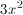if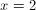.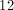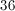Explanation: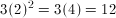### Example Question #11 : Arithmetic

Consider the function: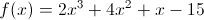What is the value of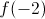?

It is impossible to square a negative number.

–49

–17

–33

17

–17

Explanation: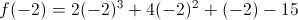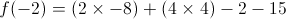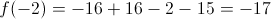### Example Question #17 : Order Of Operations

Solve: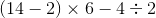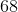Explanation:

To solve the above expression we must follow PEMDAS.

So the parentheses are first.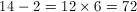.

Then we need to divide 4 by 2 before subtracting because division comes before subtraction.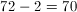### Example Question #11 : Arithmetic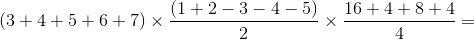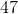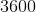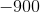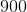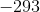Explanation:

Order of operations: "PEMDAS”, or "Parentheses, Exponents, Multiplication and Division, and Addition and Subtraction".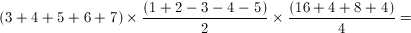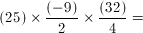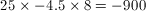### Example Question #19 : Order Of Operations

Evaluate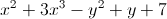when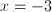and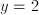.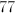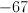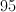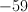None of the other answer choices are correct.Explanation:

We can evaluatewhenandby plugging in and substituting: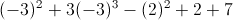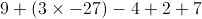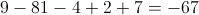### Example Question #11 : Arithmetic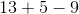When doing operations of subtraction and addition, there is no priority but we must work from left to right. The sum on the left isand we do the subtraction. The answer is.